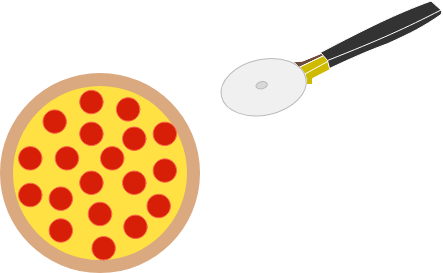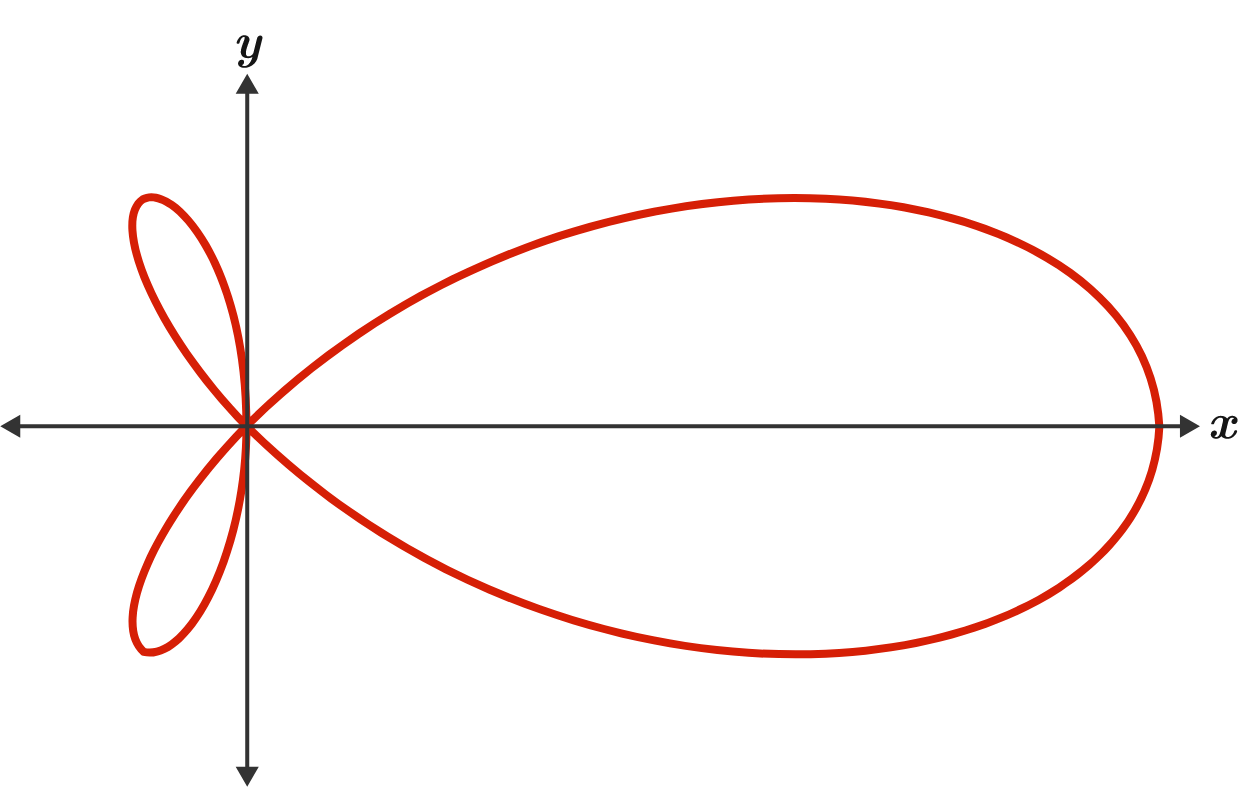# Problems of the Week

Contribute a problem

What's the minimum number of straight cuts required to cut a pizza into $7$ pieces of equal area?

Details and Assumptions:

• A straight cut may start and end anywhere on the pizza—not just on the circumference.
• The pizza comes uncut and round in the box.
• You cannot take the pizza out of the box or move any part of it.At a party with 100 people, there is a pie. The first guest gets 1% of the pie, the second gets 2% of what is left, the third gets 3% of what is left and so on.

If the $n$th guest gets the largest piece of all 100, what is $n$?

This is the graph of $r=\frac{\sin4\theta}{\sin\theta}$ in polar coordinates.

Rotating the graph about the $x$-axis produces a volume of $\frac{a}{b}\pi$, where $a$ and $b$ are coprime positive integers.

What is $a+b?$$\large \int_0^\frac \pi 2\sqrt{\tan{x}}\ dx$

Evaluate this integral.

Let $F_n$ denote the $n^\text{th}$ Fibonacci number, where $F_1 = 1, F_2 = 1,$ and $F_{n+2} = F_{n+1} + F_{n}$ for $n=1,2,3,\ldots.$

Define $f_n (x)$ as a least-degree polynomial that passes through the coordinates $(x,y)= (1, F_1), (2,F_2) , (3,F_3) , \ldots , (n-1, F_{n-1}).$ Hence, we define the expected Fibonacci sequence $^e F_n$ to be equal to $f_n (n).$

For example, with $F_1 = F_2 = 1$ and $F_3 = 2,$ we have $f_4 (x) = \frac12\big(x^2-3x+4\big),$ so $^e F_4 = f_4 (4) = 4.$

Find the closed form of the limit below (submit your answer to three decimal places): $\lim_{n\to\infty} \dfrac{ \big|^e F_n - F_n \big|}{F_n}.$

Notation: $| \cdot |$ denotes the absolute value function.

×